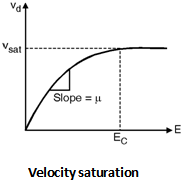Home > Digital CMOS Design > Non Ideal Effects > Velocity Saturation

Velocity-Saturation

From the physics of semiconductors it is proved that the velocity of charge carriers is linearly proportional to the electric field and the proportionality constant is called as mobility of carrier. But when we increase the electric field beyond certain velocity called as the thermal velocity or saturated velocity the velocity of the charge carrier does not change with electric field as shown in Figure below.The electric field at which the velocity of carrier saturates is called as the critical electric field. The loss of energy is because of the collisions of carriers called as scattering effect.

In MOSFETs when electrical field along the channel reaches a critical value the velocity of carriers tends to saturate and the mobility degrades. The saturation velocity for electrons and holes is approximately same i.e. 107 cm/s. The critical field at which saturation occurs depends upon the doping levels and the vertical electric field applied.

The velocity as a function of electric field plotted in Fig. 7.3.1(a) can be approximated by equation :

 = n E1 + EEc for E  Ec = sat for E  Ec

If we solve the Equations the velocity saturation effect then the modified expression for the drain current in linear region is,

IDS = n Cox1 + VDSEc L  WL (VGS  VTH) VDS  VDS22

IDS = n Cox WL (VGS  VTH) VDS  VDS22 F (VDS)

Where the factor, F (V) measures the degree of velocity saturation and is defined as :

F (V) = 11 + VEc L

VDSL is the average field in the channel. When we increase the drain to source voltage the electrical field in the channel reaches the critical value due to this the carriers at the drain are velocity saturated. Hence, the saturation drain voltage VDSSAT can be calculated by using the current at the drain.

Assuming that the drift velocity is saturated and equal to sat, the IDSSAT ,

IDSSAT = sat Cox W (VGS  VTH  VDSSAT)

= n Cox WL (VGS  VTH) VDSSAT  VDSSAT22  F(VDSSAT)

By solving this equation,

We get, VDSSAT = F (VGS  VTH)  (VGS  VTH)

= VGS  VTH1 + (VGS  VTH)Ec L

If we further increase the drain to source voltage then current will not increase and the transistor current saturates at IDSSAT.